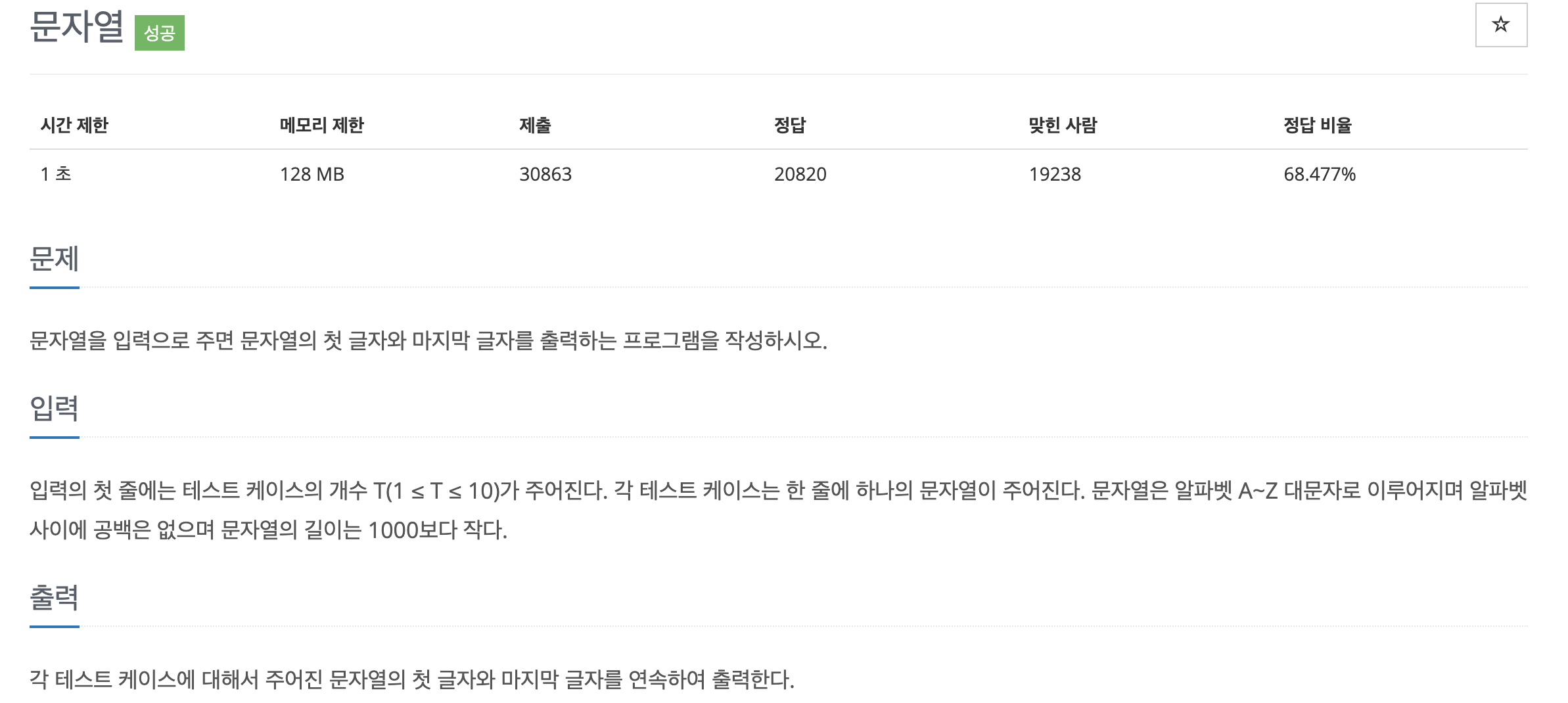# [BOJ 9086]string

kevin77774850·2023년 6월 4일
2

## BOJ

목록 보기
1/1

### problem

Write a program that prints the first and last letters of a string given a string as input.

### input

In the first line of input, the number of test cases T (1 ≤ T ≤ 10) is given. Each test case is given one string per line. The string consists of uppercase letters A to Z, there are no spaces between the letters, and the length of the string is less than 1000.

### PrintFor each test case, the first and last letters of the given string are printed consecutively.

## 1.INTUITION

Print the first and the last character of the word.

## 2.APPROACH

Input a string and print the beginning and the end by using indexing.

## 3.WHAT TO KNOW

#### 1.Input many lines

(1)Get T input and convert to integer.
(2)Using a for loop to take an input

#### 2.Indexing string

Like the list data type, string correspond to an index number. It starts at index 0.

Word  :  H   e   l   l  o     w  o  r  l  d  !
index :  0   1   2   3  4  5  6  7  8  9 10 11
index : -12 -11 -10 -9 -8 -7 -6 -5 -4 -3 -2 -1

The first H starts at index 0, and ! ends at index 11.
Also, there is an negative index. We can cound backwords from the end of the string , starting at -1.

## 4.CODE

# Get T input and convert to integer.
T = int(input())
# Using a for loop to take input.
for i in range(T):
word = input()
# print first and last chracter of the word
print(word+word[-1])